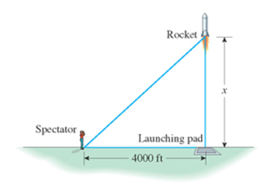Chapter 1.3, Problem 44E

Chapter
Section
Textbook Problem

Watching a Rocket Launch At a distance of 4000 ft from the launch site, a spectator is observing a rocket being launched. Suppose the rocket lifts off vertically and reaches an altitude of x ft (see the accompanying figure).a. Find an expression giving the distance between the spectator and the rocket. b. What is the distance between the spectator and the rocket when the rocket reaches an altitude of 20,000 ft?

(a)

To determine

To find: The expression of distance between the spectator and rocket.

Explanation

Given information:

A spectator observed a rocket being launch from distance of 4000 ft from launch. The rocket lifts off vertically and reaches an altitude of x ft.

Calculation:

Let the distance between spectator and rocket be d. Use Pythagoras theorem to evaluate the value of d.

The formula to calculate the distance from Pythagoras theorem is, (Hypotenuse)=(Base)2+(Perpendicular)2

(b)

To determine

To find: The distance between the spectator and rocket when rocket reaches an altitude of 20,000 ft.

Still sussing out bartleby?

Check out a sample textbook solution.

See a sample solution

The Solution to Your Study Problems

Bartleby provides explanations to thousands of textbook problems written by our experts, many with advanced degrees!

Get Started